Q-Chem 5.1 User’s Manual

# 11.12 Linear-Scaling Computation of Electric Properties

The search for new optical devices is a major field of materials sciences. Here, polarizabilities and hyperpolarizabilities provide particularly important information on molecular systems. The response of the molecular systems in the presence of an external, monochromatic, oscillatory electric field is determined by the solution of the time-dependent SCF (TDSCF) equations. Within the dipole approximation, the perturbation is represented as the interaction of the molecule with a single Fourier component of the external field,:(11.67)

with(11.68)

Here,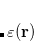is the field frequency andis the dipole moment operator. The TDSCF equations can be solved via standard techniques of perturbation theory. As a solution, one obtains the first-order perturbed density matrix [] and the second-order perturbed density matrices []. From these quantities, the following properties can be calculated:

• Static polarizability:• Dynamic polarizability:• Static hyperpolarizability:• Second harmonic generation:• Electro-optical Pockels effect:• Optical rectification:Here,is the matrix representation of thecomponent of the dipole moment.

Note that third-order properties () can be computed either with the equations above, which is based on a second-order TDSCF calculation (for), or alternatively from first-order properties using Wigner’srule. The second-order approach corresponds to MOPROP job numbers 101 and 102 (see below) whereas use of therule corresponds to job numbers 103 and 104. Solution of the second-order TDSCF equations depends upon first-order results and therefore convergence can be more problematic as compared to the first-order calculation. For this reason, we recommend job numbers 103 and 104 for the calculation of first hyperpolarizabilities.

The TDSCF calculation is more time-consuming than the SCF calculation that precedes it (where the field-free, unperturbed ground state of the molecule is obtained). Q-Chem’s implementation of the TDSCF equations is MO based and the cost therefore formally scales asymptotically as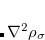. The prefactor of the cubic-scaling step is rather small, however, and in practice (over a wide range of molecular sizes) the calculation is dominated by the cost of contractions with two-electron integrals, which is formally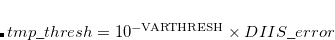scaling but with a very large prefactor. The cost of these integral contractions can be reduced from quadratic to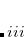using LinK/CFMM methods (Section 4.6). All derivatives are computed analytically.

The TDSCF module in Q-Chem is know as “MOProp", since it corresponds (formally) to time propagation of the molecular orbitals. (For actual time propagation of the MOs, see Section 7.11.) The MOProp module has the following features:

• LinK and CFMM support to evaluate Coulomb- and exchange-like matrices

• Analytic derivatives

• DIIS acceleration

• Both restricted and unrestricted implementations of CPSCF and TDSCF equations are available, for both Hartree-Fock and Kohn-Sham DFT.

• Support for LDA, GGA, and global hybrid functionals. Meta-GGA and range-separated functionals are not yet supported, nor are functionals that contain non-local correlation (e.g., those containing VV10).

## 11.12.1 $fdpfreq Input Section For dynamic response properties (i.e.,), various values ofmight be of interest, and it is considerably cheaper to compute properties for multiple values ofin a single calculation than it is to run several calculations for one frequency each. The$fdpfreq input section is used to specify the frequencies of interest. The format is:

$fdpfreq property frequencies units$end


The first line is only required for third-order properties, to specify the flavor of first hyperpolarizability. The options are

• StaticHyper (static hyperpolarizability)

• SHG (second harmonic generation)

• EOPockels (electro-optical Pockels effect)

• OptRect (optical rectification)

The second line in the $fdpfreq section contains floating-point values representing the frequencies of interest. Alternatively, for dynamic polarizabilities an equidistant sequence of frequencies can be specified by the keyword WALK (see example below). The last line specifies the units of the input frequencies. Options are: • au (atomic units of frequency) • eV (frequency units, expressed in electron volts) • Hz (frequency units, expressed in Hertz) • nm (wavelength units, in nanometers) • cmInv (wavenumber units,) Example 11.259 Static and dynamic polarizabilities, atomic units: $fdpfreq
0.0 0.03 0.05
au
$end  Example 11.260 Series of dynamic polarizabilities, starting with 0.00 incremented by 0.01 up to 0.10: $fdpfreq
walk 0.00 0.10 0.01
au
$end  Example 11.261 Static first hyperpolarizability, second harmonic generation and electro-optical Pockels effect, wavelength in nm: $fdpfreq
StaticHyper SHG EOPockels
1064
nm
$end  ## 11.12.2 Job Control for the MOProp Module The MOProp module is invoked by specifying a job number using the MOPROP$rem variable. In addition to electric properties, this module can also compute NMR chemical shifts (MOPROP = 1); this functionality is described in Section 11.13.

MOPROP
 Specifies the job number for MOProp module.

TYPE:
 INTEGER

DEFAULT:
 0 Do not run the MOProp module.

OPTIONS:
 1 NMR chemical shielding tensors. 2 Static polarizability. 3 Indirect nuclear spin–spin coupling tensors. 100 Dynamic polarizability. 101 First hyperpolarizability. 102 First hyperpolarizability, reading First order results from disk. 103 First hyperpolarizability using Wigner’srule. 104 First hyperpolarizability using Wigner’srule, reading first order results from disk.

RECOMMENDATION:
 None

MOPROP_PERTNUM
 Set the number of perturbed densities that will to be treated together.

TYPE:
 INTEGER

DEFAULT:
 0

OPTIONS:
 0 All at once.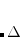Treat the perturbed densities batch-wise.

RECOMMENDATION:
 Use the default. For large systems, limiting this number may be required to avoid memory exhaustion.

MOPROP_CONV_1ST
 Sets the convergence criteria for CPSCF and 1st order TDSCF.

TYPE:
 INTEGER

DEFAULT:
 6

OPTIONS:Convergence threshold set to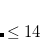.

RECOMMENDATION:
 None

MOPROP_CONV_2ND
 Sets the convergence criterion for second-order TDSCF.

TYPE:
 INTEGER

DEFAULT:
 6

OPTIONS:Convergence threshold set to.

RECOMMENDATION:
 None

MOPROP_MAXITER_1ST
 The maximum number of iterations for CPSCF and first-order TDSCF.

TYPE:
 INTEGER

DEFAULT:
 50

OPTIONS:Set maximum number of iterations to.

RECOMMENDATION:
 Use the default.

MOPROP_MAXITER_2ND
 The maximum number of iterations for second-order TDSCF.

TYPE:
 INTEGER

DEFAULT:
 50

OPTIONS:Set maximum number of iterations to.

RECOMMENDATION:
 Use the default.

MOPROP_ISSC_PRINT_REDUCED
 Specifies whether the isotope-independent reduced coupling tensorshould be printed in addition to the isotope-dependent-tensor when calculating indirect nuclear spin-spin couplings.

TYPE:
 LOGICAL

DEFAULT:
 FALSE

OPTIONS:
 FALSE Do not print. TRUE Print.

RECOMMENDATION:
 None

MOPROP_ISSC_SKIP_FC
 Specifies whether to skip the calculation of the Fermi contact contribution to the indirect nuclear spin-spin coupling tensor.

TYPE:
 LOGICAL

DEFAULT:
 FALSE

OPTIONS:
 FALSE Calculate Fermi contact contribution. TRUE Skip Fermi contact contribution.

RECOMMENDATION:
 None

MOPROP_ISSC_SKIP_SD
 Specifies whether to skip the calculation of the spin-dipole contribution to the indirect nuclear spin-spin coupling tensor.

TYPE:
 LOGICAL

DEFAULT:
 FALSE

OPTIONS:
 FALSE Calculate spin-dipole contribution. TRUE Skip spin-dipole contribution.

RECOMMENDATION:
 None

MOPROP_ISSC_SKIP_PSO
 Specifies whether to skip the calculation of the paramagnetic spin-orbit contribution to the indirect nuclear spin-spin coupling tensor.

TYPE:
 LOGICAL

DEFAULT:
 FALSE

OPTIONS:
 FALSE Calculate paramagnetic spin-orbit contribution. TRUE Skip paramagnetic spin-orbit contribution.

RECOMMENDATION:
 None

MOPROP_ISSC_SKIP_DSO
 Specifies whether to skip the calculation of the diamagnetic spin-orbit contribution to the indirect nuclear spin-spin coupling tensor.

TYPE:
 LOGICAL

DEFAULT:
 FALSE

OPTIONS:
 FALSE Calculate diamagnetic spin-orbit contribution. TRUE Skip diamagnetic spin-orbit contribution.

RECOMMENDATION:
 None

MOPROP_DIIS
 Controls the use of Pulay’s DIIS in solving the CPSCF equations.

TYPE:
 INTEGER

DEFAULT:
 5

OPTIONS:
 0 Turn off DIIS. 5 Turn on DIIS.

RECOMMENDATION:
 None

MOPROP_DIIS_DIM_SS
 Specified the DIIS subspace dimension.

TYPE:
 INTEGER

DEFAULT:
 20

OPTIONS:
 0 No DIIS.Use a subspace of dimension.

RECOMMENDATION:
 None

SAVE_LAST_GPX
 Save the lastwhen calculating dynamic polarizabilities in order to call the MOProp code in a second run, via MOPROP = 102.

TYPE:
 INTEGER

DEFAULT:
 0

OPTIONS:
 0 False 1 True

RECOMMENDATION:
 None

MOPROP_RESTART
 Specifies the option for restarting MOProp calculations.

TYPE:
 INTEGER

DEFAULT:
 0

OPTIONS:
 0 Not a restart calculation. 1 Restart from a previous calculation using the same scratch directory.

RECOMMENDATION:
 Need to also include "SCF_GUESS READ" and "SKIP_SCFMAN TRUE" to ensure the same set of MOs.

## 11.12.3 Examples

Example 11.262B97X-D/def2-SVPD static polarizability calculation for water cation, computed analytically using the MOProp module

$rem method hf basis def2-svpd scf_convergence 11 thresh 14 symmetry false sym_ignore true moprop 2 moprop_conv_1st 8 moprop_maxiter_1st 200$end

$molecule 1 2 O 0.003 1.517 0.000 H 0.913 1.819 0.000 H 0.081 0.555 0.000$end